Number Words - Definition with Examples

The Complete K-5 Math Learning Program Built for Your Child

• 30 Million Kids

Loved by kids and parent worldwide

• 50,000 Schools

Trusted by teachers across schools

• Comprehensive Curriculum

Aligned to Common Core

Number words

Number words are the alphabetical form of numbers. As the name suggests, these are numbers written in words.

For example: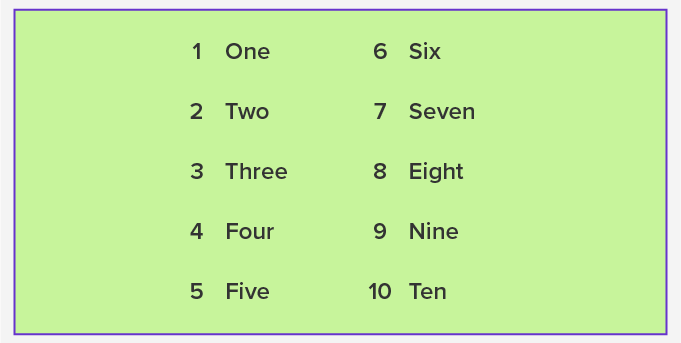With the help of the number words from one to ten, we can make number words of higher value.

It is important to learn every number word from One to Twenty to learn other number words. Also, the number words from eleven to twenty are very different from other number words.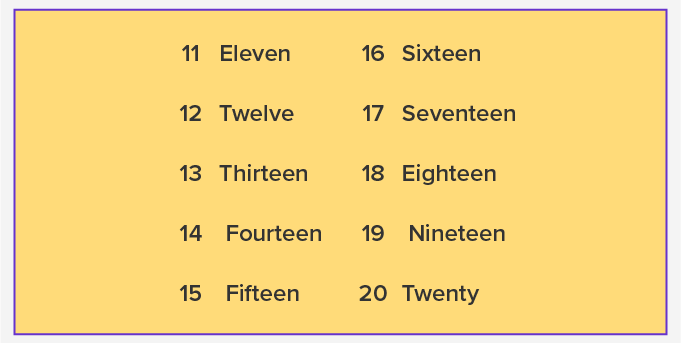After learning the number words up to twenty, it is important to learn the number words such as thirty, forty, fifty, sixty until hundred.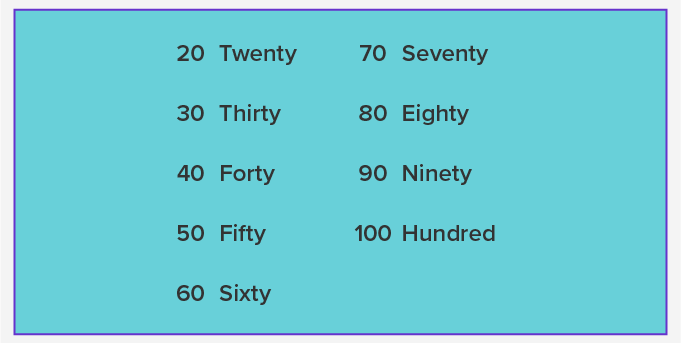Once you know these number words, it is easy to make number words for higher value numbers.

For example: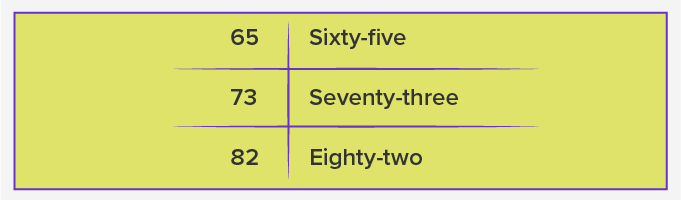The above two number words are made from learning the basic number words from one to ten, and the number words for tens value, like sixty, seventy and eighty.

As the numbers increase in value and become larger with three, four, five, six, seven and more digits, the names start to change.

The numbers for higher values are: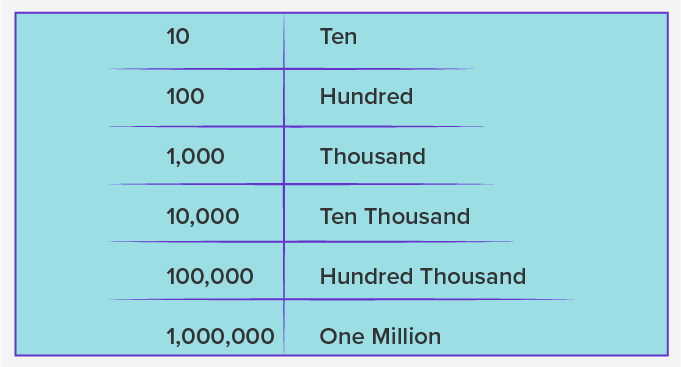It becomes easy to convert numbers to number words and number words to number if we know the number words.

For example:

Write the given numbers in words.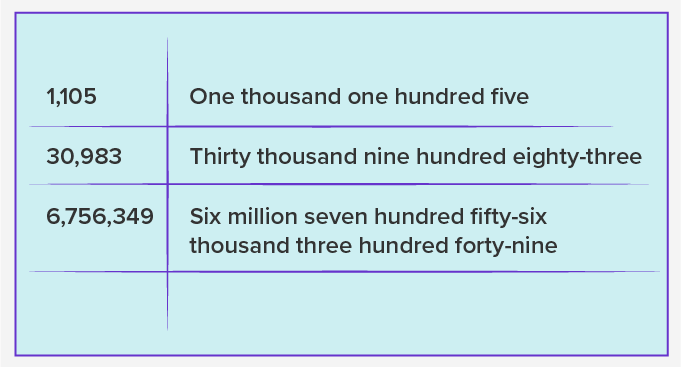Write the given numbers in numerals.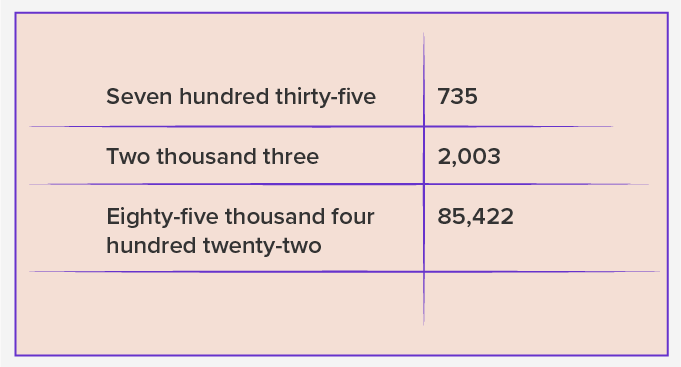Fun Facts The number word for 40 is spelled as Forty and not Fourty. To write number words, we can write the word form of the numbers as we say them.

Won Numerous Awards & Honors